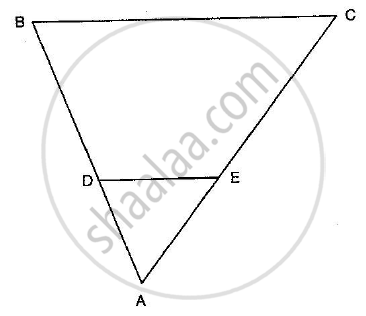Advertisement Remove all ads

# In the Given Figure, De || Bc in ∆Abc Such that Bc = 8 Cm, Ab = 6 Cm and Da = 1.5 Cm. Find De. - Mathematics

Sum

In the given figure, DE || BC in ∆ABC such that BC = 8 cm, AB = 6 cm and DA = 1.5 cm. Find DE.Advertisement Remove all ads

#### Solution

Given: In ∆ABC, DE || BC. BC = 8 cm, AB = 6 cm and DA = 1.5 cm.

To find: DE

In ∆ABC and ∆ADE

$\angle B = \angle ADE \left( \text{Corresponding angles} \right)$
$\angle A = \angle A \left( \text{Common} \right)$
$\therefore ∆ ABC~ ∆ ADE \left( \text{AA Similarity} \right)$

so,

(BC)/(DE)=(AB)/(DA)

8/(DE)=6/1.5

DE= (8xx1.5)/6

DE= 2cm

Concept: Triangles Examples and Solutions
Is there an error in this question or solution?
Advertisement Remove all ads

#### APPEARS IN

RD Sharma Class 10 Maths
Chapter 7 Triangles
Q 20 | Page 130
Advertisement Remove all ads

#### Video TutorialsVIEW ALL 

Advertisement Remove all ads
Share
Notifications

View all notifications

Forgot password?
Course